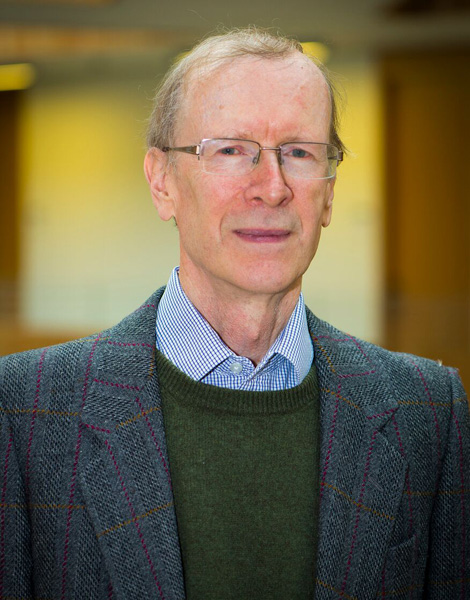# Abel Prize given for proof of Last Theorem

Sir Andrew J. Wiles is recognized for proving one of the most difficult challenges in mathematics: Fermat’s Last TheoremPhoto courtesy of the Norwegian Academy
of Science and Letters
Sir Andrew J. Wiles.

This year the Norwegian Academy of Science and Letters awarded the Abel Prize to British mathematician Sir Andrew J. Wiles for a proof of Fermat’s Last Theorem, first proposed in 1637 by French mathematician Pierre de Fermat (1601-1665) and first successfully solved in 1995 by Wiles.

In everyday language, that Fermat’s Last Theorem went unsolved for 358 years shows that in the precise world of mathematics, generalizations of simple truths sometimes are devilishly difficult to prove. Consider the Pythagorean Theorem that we learn about in high-school geometry. It states that the square of the hypotenuse of a right triangle is equal to the sum of the squares of the other two sides. Expressed in an equation, where a and b are the lengths of the sides and c is the length of the hypotenuse, it is:

a2 + b2 = c2

The right triangle often is called the “3-4-5 triangle” because the integers 3, 4, and 5 are the lowest ones for which the equation is valid: 3 squared plus 4 squared equals 5 squared: 9 + 16 = 25. That simple truth suggests that there might be a generalization of the equation. Fermat’s Conjecture, now called his “Last Theorem” says there isn’t. The equation

an + bn = cn

has no solution in positive integers if the exponent n is an integer greater than 2. Fermat claimed to have worked out a proof of the conjecture but left no details of it. For three and a half centuries, Fermit’s Last Theorem stood unproven in mathematics. In the Guinness Book of World Records it ranked as the most difficult challenge of mathematics, in part because it had the greatest number of unsuccessful proofs.

The magnitude of the challenge was staggering. Mathematician Wiles spent seven years of research time in compiling his successful 150-page proof of Fermat’s Last Theorem. For that achievement he was knighted in 2000 and this year is the Abel Prize laureate.
The prize commemorates the significant contributions of Norwegian mathematician Nils Henrik Abel (1802-1829). It was first proposed in 1899 by Norwegian mathematician Sophus Lie (1842-1899) when he learned that Swedish industrialist Albert Nobel’s plan for annual prizes did not include a prize in mathematics. With Lie’s death in 1899 and the dissolution of the Union between Norway and Sweden in 1905, his proposal of an Abel Prize in mathematics was shelved.

But in 2001 Lie’s proposal was revived, and the Norwegian government announced that a mathematics prize would be awarded annually starting in 2002, the bicentennial of the birth of mathematician Abel. Now known as the Abel Prize, it complements the Holberg Prize in the humanities and comes with a monetary award of NOK 6 million (\$721,000). The 2016 award to mathematician Wiles was made on May 24 by HRH Crown Prince Haakon at a ceremony in the University Aula in Oslo.

M. Michael Brady was educated as a scientist and with time turned to writing and translating.

This article originally appeared in the June 3, 2016, issue of The Norwegian American. To subscribe, visit SUBSCRIBE or call us at (206) 784-4617.The Norwegian American

Published since May 17, 1889 PO Box 30863 Seattle WA 98113 Tel: (206) 784-4617 • Email: naw@na-weekly.com